## ML Aggarwal Class 8 Solutions for ICSE Maths Chapter 7 Percentage Ex 7.2

Question 1.
Find the profit or loss percentage, when :
(i) C.P. = ₹400, S.P. = ₹468
(ii) C.P. = ₹ 13600, S.P. = ₹12104
Solution:
(i) C.P. = ₹400, S.P. = ₹468
Profit = S.P. – C.P. = 468 – 400 = ₹68(ii) C.P. = ₹13600, S.P. = ₹12104
Loss = C.P. – S.P.
= ₹13600 – ₹12104 = ₹1496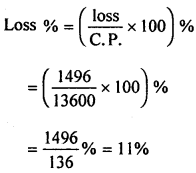Question 2.
By selling an article for ₹1636·25, a dealer gains ₹ 96·25. Find his gain per cent.
Solution:
S.P. of an article = ₹1636·25
Gain = ₹96·25
C.P. = S.P. – Gain
= ₹1636·25 – ₹96·25
= ₹1540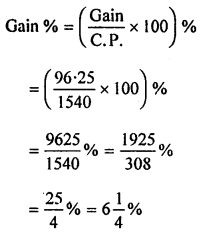Question 3.
By selling an article for ₹770, a man incurs a loss of ₹110. Find his loss percentage.
Solution:
S.P. of an article = ₹ 770
Loss = ₹ 110
C.P. = S.P. + Loss = ₹ 770 + ₹ 110 = ₹ 880Question 4.
Rashida bought 25 dozen eggs at the rate of ₹9.60 per dozen. 30 eggs were broken in the transaction and she sold the remaining eggs at one rupee each. Find her gain or loss percentage.
Solution:
C.P. of one dozen eggs = ₹ 9.60
C.P. of 25 dozen eggs = ₹ 25 × 9.60 = ₹ 240
Number of eggs = 25 dozen = 25 × 12 = 300
Number of eggs broken in transaction = 30
Number of remaining eggs = 300 – 30 = 270
S.P. of one egg = ₹ 1
S.P. of 270 eggs = ₹ 1 × 270 = ₹ 270
Profit = S.P. – C.P. = ₹ 270 – ₹ 240 = ₹ 30Question 5.
The cost of an article was ₹20000 and ₹1400 were spent on its repairs. If it is sold for a profit of 20%, find the selling price of the article.
Solution:
Cost of an article = ₹20000
Cost of its repair = ₹1400
Total cost = ₹20000 + ₹1400 = ₹21400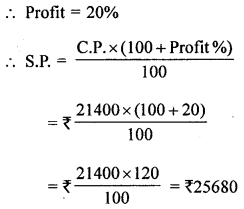Question 6.
A shopkeeper buys 200 bicycles at ₹1200 per bicycle. He spends ₹30 per bicycle on transportation. He also spends ₹4000 on advertising. Then he sells all the bicycles at ₹1350 per piece. Find his profit or loss. Also, calculate it as a percentage.
Solution:
Cost price of one bicycle = ₹1200
Cost price of 200 bicycle = ₹1200 × 200 = ₹240000
Expenditure on transportation for one bicycle = ₹30
Expenditure on transportation for 200 bicycle
= ₹30 × 200 = ₹6000
Net C.P. of the bicycle
= ₹240000 + ₹6000 + ₹4000 = ₹250000
S.P. of 200 bicycle at ₹1350 per bicycle
= ₹200 × 1350 = ₹270000
Profit = S.P. – C.P. = ₹270000 – ₹250000 = ₹20000Question 7.
The cost price of an article is 90% of its selling price. Find his profit percentage.
Solution:
Let S.P. of an article = ₹ x
Then C.P. of an article = 90 % of ₹ xQuestion 8.
Rao bought notebooks at the rate of 4 for ₹35 and sold them at the rate of 5 for ₹58. Calculate
(i) his gain percentage.
(ii) the number of notebooks he should sell to earn a profit of ₹171.
Solution:
Let the number of note books bought = 20
[Note : L.C.M. of 4 and 5 = 20]
∴ C.P. of the note books = ₹$$\frac{35}{4}$$ × 20 = ₹ 35 × 5 = ₹ 175
and S.P. of the note books = ₹$$\frac{58}{5}$$ × 20 = ₹58 × 4 = ₹232
(i) Gain = S.P. – C.P. = ₹ 232 – ₹175 = ₹57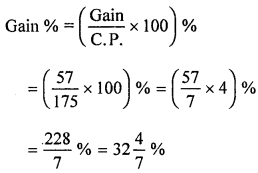(ii) When profit is ₹57, the quantity of note books sold = 20
When profit is ₹1, the quantity of note books sold = $$\frac{20}{57}$$
When profit is ₹171, the quantity of note books sold = $$\frac{20}{57}$$ × 171
= 20 × 3 = 60

Question 9.
A vendor buys bananas at 3 for a rupee and sells at 4 for a rupee. Find his profit or loss percentage.
Solution:
Let number of bananas bought = 12
[Note : L.C.M. of 3 and 4 = 12]
∴ C.P. of bananas = ₹ $$\frac{1}{3}$$ × 12 = ₹4
and S.P. of bananas = ₹$$\frac{1}{4}$$ × 12 = ₹3
Loss = C.P. – S.P. = ₹4 = ₹3 = ₹1
Loss % = $$\left(\frac{\text { Loss }}{\text { C.P. }} \times 100\right) \%$$
= $$\left(\frac{1}{4} \times 100\right) \%$$
= $$\frac{100}{4} \%=25 \%$$

Question 10.
A shopkeeper buys a certain number of pens. If the selling price of 5 pens is equal to the cost price of 7 Pens, find his profit or loss percentage.
Solution:
Let the cost price of 7 Pens = ₹ x
Cost price of 1 Pen = ₹ $$\frac{x}{7}$$
Also, according to question,
Selling price of 5 Pens = ₹x
Selling price of 1 Pens = ₹ $$\frac{x}{5}$$
Profit = S.P. – C.P. = ₹ $$\frac{x}{7}$$ – ₹ $$\frac{x}{5}$$Question 11.
Find the selling price, when :
(i) Cost price = ₹2360, Profit = 8%
(ii) Cost price = ₹380, Loss = 7·5%
Solution:
(i) Cost price = ₹2360, Profit = 8%
We know that,
S.P = $$\left(\frac{100+\text { Profit } \%}{100}\right)$$ × C.P.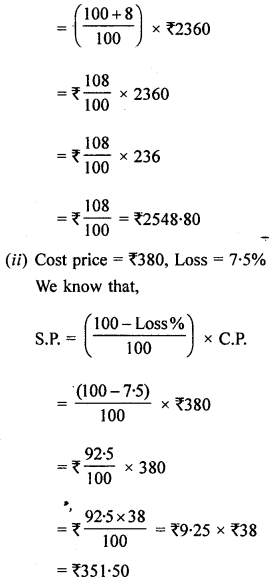Question 12.
A dealer bought a number of eggs at ₹18 a dozen and sold them at 50% profit. Find the selling price per egg.
Solution:
C.P. of one dozen eggs i.e. 12 eggs = ₹18
Profit = 50%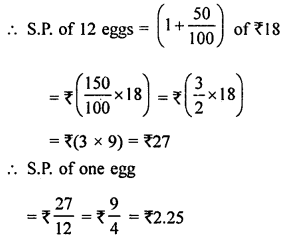Question 13.
Mr Ghosh purchased wristwatches worth ₹60000. He sold one-third of them at a profit of 30%, one-third at a profit of 20% and remaining at a loss of 5%. Calculate his overall profit or loss percentage.
Solution:
C.P. of wristwatches = ₹60000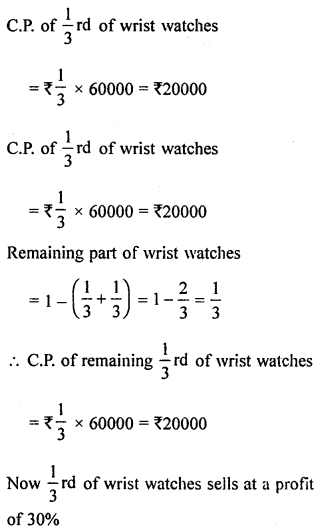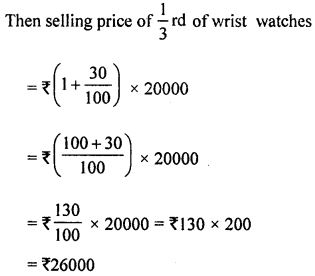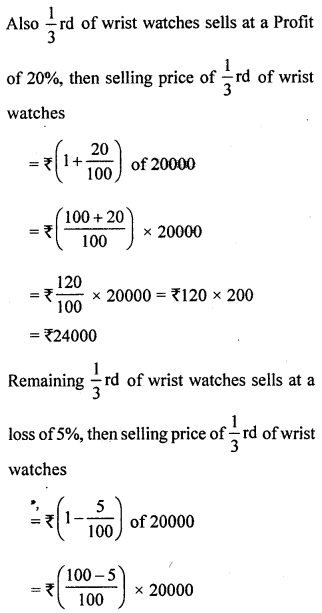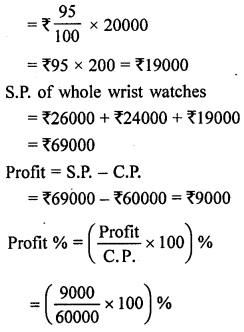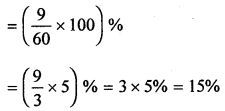Hence Profit = 15 %

Question 14.
A laptop and a mobile phone were bought for ₹40000 and ₹24000 respectively. The shopkeeper made a profit of 8% on the laptop and a loss of 12% on the mobile phone. Find his gain or loss per cent on the whole transaction.
Solution:
C.P. of laptop = ₹40000
and C.P. of mobile phone = ₹24000
Profit on laptop = 8%
and loss on mobile phone = 12%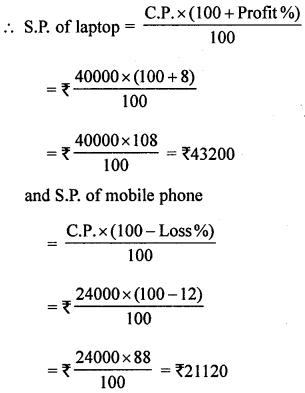Total cost = ₹40000 + ₹24000 = ₹64000
and Total S.P. = ₹43200 + ₹21120 = ₹64320
∴ Gain = S.P. – C.P. = ₹64320 – ₹64000 = ₹320
∴ Gain % = $$\frac { Gain\times 100 }{ C.P. }$$
= $$\frac{320 \times 100}{64000}=\frac{1}{2} \%$$ = 0.5%

Question 15.
Salman bought 40 chairs at ₹175 each fourth of them at a loss of 8%. At what price each must he sell the remaining chairs so as to gain 10% on the whole deal?
Solution:
C.P. of one chair = ₹175
C.P. of 40 chair = ₹175 × 40 = ₹7000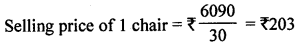Question 16.
A shopkeeper sold two electronic gadgets for ₹44000 each. The shopkeeper made a loss of 12% on one and a profit of 10% on the other. Find his overall gain or loss.
Solution:
S.P. of first gadget = ₹44000
Loss = 12%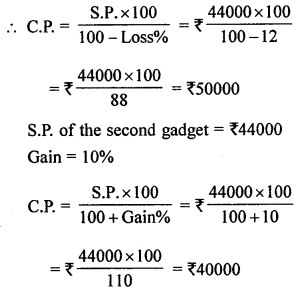Now S.P. of both gadgets = ₹44000 × 2 = ₹88000
Their cost price = ₹50000 + ₹40000 = ₹90000
∴ Loss = C.P. – S.P. = ₹90000 – ₹88000 = ₹2000
Overall loss = ₹2000

Question 17.
The manufacturing price of a T.V. set is ₹12000. The company sold it to a dealer at 20% profit and the dealer sold it to a customer at 12·5% profit. Find the price which the customer has to pay.
Solution:
The manufacturing price of a T.V. set = ₹12000
S.P. for company = ₹ $$\left(1+\frac{20}{100}\right)$$ × 12000Question 18.
Find the cost price, when :
(i) selling Price = ₹450, loss = 10%
(ii) selling Price = ₹690, profit = 15%
Solution:
(i) Selling price = ₹450, loss =10%
we know that,∴ C.P. = ₹5 × 100 = ₹500
(ii) Selling price = ₹690, Profit = 15%
We know that,⇒ C.P. = ₹6 × 100 = ₹600

Question 19.
By selling an almirah for ₹3920, a shopkeeper would gain 12%. If it is sold for ₹4375, find his gain or loss, percentage.
Solution:
When Selling price of almirah = ₹3920
and Gain % = 12%
then C.P. =?
We know that,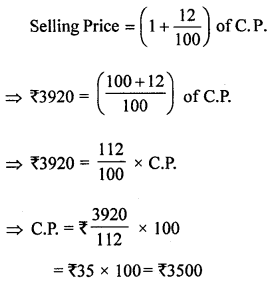Now when C.P. of almirah = ₹3500
and S.P. of almirah = ₹4375
gain = S.P. – C.P. = ₹4375 – ₹3500 = ₹875Question 20.
By selling a bicycle at ₹1334, a shopkeeper would suffer a loss of 8%. At how much amount should he sell it to make a profit of $$12 \frac{1}{2}$$%?
Solution:
When selling price of bicycle = ₹1334
Loss % = 8%
C.P. = ?
We know that,
Selling price = (1 – loss%) of C.P.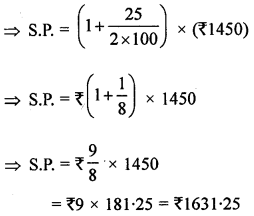Question 21.
By selling a tie for ₹252, a shopkeeper gains 5%. At what price should he sell the tie to gain 35% ?
Solution:
Selling price of tie = ₹252
gain% = 5%
C.P. = ?
We know that,
Selling price = (1 + Gain %) of C.P.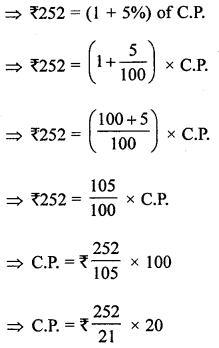⇒ C.P. = ₹12 × 20 = ₹240
Now C.P. = ₹240
If shopkeeper wants a gain of 35%,
then Selling price = (1 + gain %) of C.P.Question 22.
A shopkeeper sells a bag at a 12% profit. If he had sold it for ₹39 more, he would have made 18% profit. Find the cost price of the bag for the shopkeeper.
Solution:
First time gain = 12%
and second time gain = 18%
∴ Difference in gain % = 18 – 12 = 6%
Actual difference = ₹39
If gain is ₹6, then C.P. = ₹100
and if gain is ₹1, then C.P. = $$\frac{100}{6}$$
and if gain is ₹39. then C.P. = ₹ $$\frac{100 \times 39}{6}$$ = ₹650

Question 23.
A shopkeeper sells a sweater at a loss of 5%. If he had sold it for ₹260 more, he would . have made a profit of 15%. Calculate the
purchase price of the sweater.
Solution:
Let the cost price of sweater = ₹ x
Loss = 5%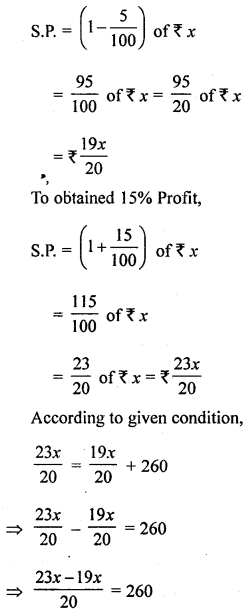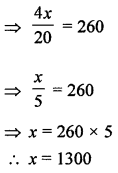Hence the cost price of sweater = ₹1300

Question 24.
Janki sold her leather purse at 8% loss. If she had sold it for ₹ 150 more, she would have made 12% profit. Find the selling price of the purse.
Solution:
Let the selling price leather purse = ₹ x
Loss = 8%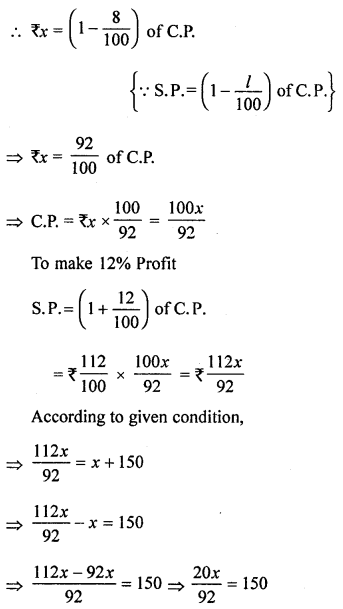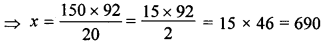Hence the selling price of leather purse = ₹690